# 9.4   Numerical Solutions to Differential Equations

This section under major construction.

Solving differential equations is a fundamental problem in science and engineering. A differential equation is ... For example: y' = -2y, y(0) = 1 has an analytic solution y(x) = exp(-2x). Laplace's equation d2φ/dx2 + d2φ/dy2 = 0 plus some boundary conditions. Sometimes we can find closed-form solutions using calculus. However, in general we must resort to numerical approximations. ODE = differential equation in which all dependent variables are a function of a single independent variable, as in the first example. PDE = differential equation in which all dependent variables are a function of several independent variables, as in the second example.

Euler method. In the 18th century Leonhard Euler invented a simple scheme for numerically approximating the solution to an ODE. Given a first order ODE of the form dy/dx = f(x, y) subject to the initial boundary condition y(x0) = y0, we estimate the function y(x) over a regular sample of values xn = x0 + hn. The parameter h is referred to as the step length. If yn is the approximation to y(x) at xn, then we can approximate the gradient at xn by f(xn, yn). We estimate yn+1 by assuming the gradient remains constant in the interval between xn and xn+1. This leads to the following method: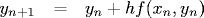The smaller we make h, the more accurate the approximation. But this comes at the expense of more computation. The associated truncation error can be formalized by comparing the above estimate with the Taylor series approximation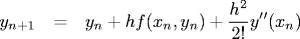We see that the truncation error is approximately O(h2). If the errors are cumulative (conservative assumption), then the total error from x0 = 0 to xn = 1 is O(h). Called a first-order method. To keep relative error below 1E-6, we must do 1 million steps. Note if the step size is too big, then Euler's method becomes unstable.

Lorenz attractor. The Lorenz equations are the following system of differential equations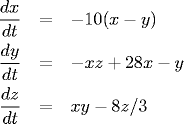Program Butterfly.java uses Euler method's to numerically solve Lorenz's equation and plots the trajectory (x, z).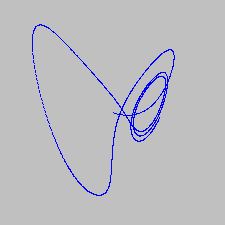Program Lorenz.java plots two trajectories of Lorenz's equation with slightly different initial conditions. This perturbation eventually leads to significantly different behavior. This is the origin of the so-called butterfly effect. Here's a good demonstration.

Runge-Kutta method. Euler's method not used in pratice because truncation error per step is relatively large compared with other methods. Also Euler's method becomes unstable if step size is too large. Euler's method only uses first derivative information at beginning of step. Runge-Kutta method samples derivative at several points in interval. Tradeoff between computing the function f(x, y) and increased accuracy. The 4th order Runge-Kutta method is a popular sweet spot.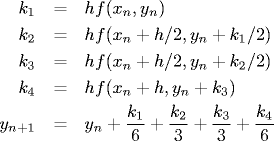It's a fourth order method.

N-body simulation. Sir Isaac Newton formulated the principles governing the the motion of two particles under the influence of their mutual gravitational attraction in his famous Principia in 1687. However, Newton was unable to solve the problem for three or more particles. Indeed, systems of three or more particles can only be solved numerically. We will describe a classic numerical solution that is widely used to study complex physical systems in cosmology, plasma physics, semiconductors, fluid dynamics and astrophysics. Scientists also apply the same techniques to other pairwise interactions including Coulombic, Biot-Savart, and van der Waals.

We first review Newton's mathematical model describing the movement of the planets. Newton's law of universal gravitation asserts that the strength of the gravitational force between two particles is given by F = Gm1m2 / R2, where G is the universal gravitational constant, m1 and m2 are the masses of the two particles, and R is the distance between them. The force is vector quantity, and the pull of one particle towards another acts on the line between them. Newton's second law of motion F = ma relates the force to the acceleration. Let mi denote the mass of particle i and let ri denote its position vector (as a function of time t). Combining Newton's two laws, we obtain a system of differential equations which characterize the motion of the particles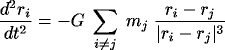Since a particle's acceleration only depends on the positions of other particles and not of their velocities (as might be the case of particles in an electromagnetic field), we can use a specialized numerical integration method known as the leapfrog finite difference approximation scheme. This is the basis for most astrophysical simulations of gravitational systems. In this scheme, we discretize time, and increment the time variable t in increments of the time quantum dt. We maintain the position and velocity of each particle, but they are half a time step out of phase (which explains the name leapfrog). The steps below illustrate how to evolve the positions and velocities of the particles. Here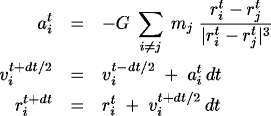James M. Stone, Astrophysics, Princeton University "Some of the most basic facts we have discovered about the Universe (for example, the temperature at the center of the Sun) are known only through the application of computational methods."

Elliptic partial differential equations. An elliptical partial differential equations involves second derivatives of space, but not time. One of big challenges in scientific computing is fast multipole methods for solving elliptic PDEs. One of the simplest and most important examples is Laplace's equation: d2φ/dx2 + d2φ/dy2 = 0. It arises in many scientific applications to model electric, gravitational, and fluid potentials. It describes the steady state of heat transport, steady state water table elevation in an unconfined aquifer, We only know values on the boundary, so no initial conditions, and Euler method doesn't apply. To solve it numerically, we can use a finite-different method on a square grid.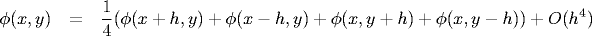Voltage at a point is the average of its nearest neighbors. Called the relaxation algorithm or Gauss-Seidel. Program Laplace.java numerically solves Lapace's equation in the plane with a fixed potential at its four boundary walls: left (30), right (70), upper (100), and bottom (0). At each step, we make each cell be the average of its four neighbors. We always use the most up-to-date value (not necessarily the same as the one from the previous step). We plot all points using an integer scale of 0 (blue) to 100 (red). We plot points with potentials equal to a multiple of 10 in white to highlight the equipotential lines. Note that because of averaging property, maximum and minimum are achieved at boundary points. The picture below illustrates the potential after 0, 500, 1,000, and 10,000 iterations. Convergence is slow.Slow convergence. The bottleneck is drawing to the screen. To speed things up, we perform 100 updates before redrawing to the screen using Turtle graphics. The method can also be sped up by choosing better estimates for the initial potentials (instead of choosing an aribtrary value).

Other techniques: solve linear system of equations. Typically involve large but, sparse and banded matrices. In 1D the finite difference scheme for Laplace's equation yields a tridiagonal system of linear equations.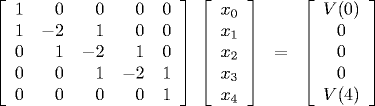Easily parallelized variants: Jacobi iteration, successive over relaxation, red-black ordering. In 2D use multigrid methods (discretized grids on several scales so that information propagates faster), conjugate gradient, or FFT if periodic boundary.

#### Q + A

Q. Why use the leapfrog method instead of Euler's method or Runge-Kutta?

A. The leapfrog method is more stable for integrating Hamiltonian systems. It is symplectic, which means it preserves properties specific to Hamiltonian systems (conservation of linear and angular momentum, time-reversibility, and conservation of energy of the discrete Hamiltonian). In contrast, ordinary numerical methods become dissipative and exhibit incorrect long-term behavior.

Q. Any good sources on numerics in Java

A. Try Java numerics.

#### Exercises

1. (example suggested by Tamara Broderick) Write a program LaplaceSquare.java to solve Laplace's equation with a fixed potential of 0 on the boundary of the grid and an internal square (of 1/9 the area) in the center with fixed potential 100.
2. Solve Laplace's equation with an L-shaped internal boundary.

#### Creative Exercises

1. Skydiver. Suppose the descent of a skydiver is modeled with the following differential equation: d2x/dt2 = 9.8 - 0.01 (dx/dt)2, where x is the distance from the drop zone (meters). Initially x(0) = 0 and x'(0) = 0. Estimate distance traveled after 3 seconds. Estimate terminal velocity. use dt = 1.
2. Poisson's equation. Poisson's equation is: dφ/dx2 + dφ/dy2 = f(x, y).
3. SIR epidemiology model. The SIR model measures the number of susceptible, infected, and recovered individuals in a host population. Given a fixed population, let S(t) be the fraction that is susceptible to an infectious, but not deadly, disease at time t; let I(t) be the fraction that is infected at time t; and let R(t) be the fraction that has recovered. Let β be the rate at which an infected person infects a susceptible person. Let γ be the rate at which infected people recover from the disease. The differential equations describing the fraction of the population susceptible, infected, and recovering people is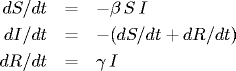The first equation models the rate of infection; the second says that the population is closed; the third models the rate of recovery. Epidemiologists use this simple model to track the infection rate of measles and ebola. Hong Kong flu: initially 7.9 million people, 10 infected, 0 recovered. Thus S(0) = 1, I(0) = 1.27E-6, R(0) = 0. Estiamte average period of infection as 3 days, so γ = 1/3 and infection rate as one new person every days, so β = 1/2. Plot S(t), I(t), and R(t) in different colors for t = 0 to 200.

Small pox: 1/γ = 14 days, 1/μ = 27375 days, 1/β = 27389/20. Corn blight: 1/γ = 20 days, 1/μ = 60 days, 1/β = 80/5.

4. Logistic map. Write a program LogisticMap.java that plots the bifurcation diagram of the logistic map. The logistic equation is : yn+1 = 4 r yn (1 - yn). For each value of r between 0.7 and 1.0, initialize y0 = 0.5, perform 1,000 iterates and discard them, then plot the next 100 iterates on the y-axis.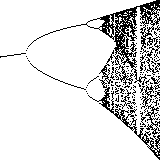5. ODE Initial value problem in chemical engineering. equations governing a fermentor.
6. Predator-prey dynamics. In the Lotka-Volterra model, there is one populations of animals (predator) that feeds on another population of animals (prey). Here is some data that approximates the populations of lynx and snowshoe hares observed by the Hudson Bay Company beginning in 1852. The x_t denote the number of snow hares (prey) and y_t be the number of lynxes (predator) living at time t. Snow hares are vegetarians and we assume the number of hares born is proportional (with birth rate α) to the number of hares alive at time t. The probability of a decisive encounter between a lynx and a hare is proportional (with kill rate β) to the product of the number of hares and the number of lynxes. We assume the lynxes have no natural enemies and the number of deaths is proportional (with birth rate γ). We also assume that the number of lynxes born is proportional to the number of hares

 dx/dt = α x - β x y dy/dt = γ x y - δ y 

Equilibrium: (0, 0) or (δ / γ, α / β). Not stable. α = 1, &beta = 0.01, γ = 0.02, δ = 1.

x_0 = 20, y_0 = 20.

1. Use Euler's method.
2. Use 4th order Runge Kutta method.